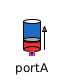Product - MapleSim Help

Complex Product

Output product of the two inputsDescription The Complex Product (or Product) component computes the product of the two complex inputs ${u}_{1}$ and ${u}_{2}$.Equations $y={u}_{1}{u}_{2}$Connections

 Name Description Modelica ID ${u}_{1}$ Complex input signal 1 u1 ${u}_{2}$ Complex input signal 2 u2 $y$ Complex output signal yParameters

 Name Default Units Description Modelica ID Use Conjugate Input 1 $\mathrm{false}$ If true, use the complex conjugate of input 1 useConjugateInput1 Use Conjugate Input 2 $\mathrm{false}$ If true, use the complex conjugate of input 2 useConjugateInput2Modelica Standard Library The component described in this topic is from the Modelica Standard Library. To view the original documentation, which includes author and copyright information, click here.